# Equivalent Fractions (3.NF.3B)

##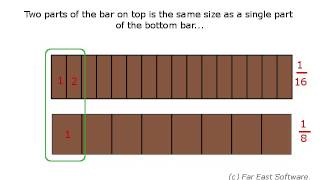By Far East Software

Recognize and generate simple equivalent fractions, e.g., 1/2 = 2/4, 4/6 = 2/3. Explain why the fractions are equivalent, e.g., by using a visual fraction model.# Equivalent Fractions

##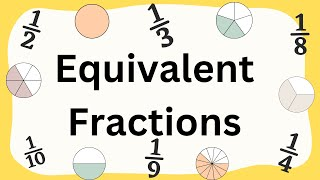By MooMoo Math and Science

defines equivalent fractions# Finding equivalent fractions with visual fraction models

##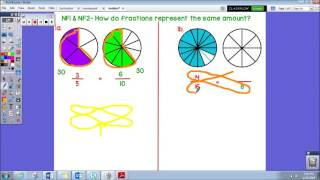By Amy Long

This video explains how to find an equivalent fraction using visual fraction models and also using cross multiplication.# Nested fractions

##By Khan Academy

Sal starts with a simple case of multiplying two fractions and proceeds to simplifying a nested fraction expression.# Equivalent Fractions on a Number Line

##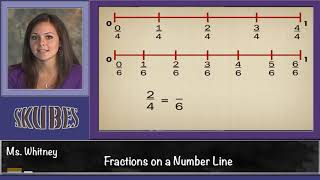By Skubes ed

Explain equivalence of fractions in special cases, and compare fractions by reasoning about their size.# Visualizing equivalent fractions | Fractions | Pre-Algebra | Khan Academy

##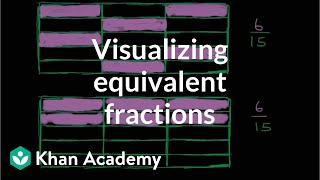By Khan Academy

To understand equivalent fractions it helps to see the fractions using something like a grid with shaded and unshaded sections representing the fraction.# Comparing Fractions

##By Jason Hall

Compare two fractions with different numerators and different denominators# Fractions to decimals

##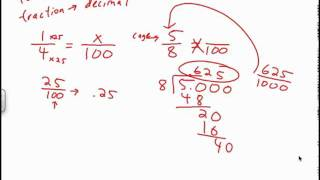By Mathademics

How to write a fraction as a decimal.# Types Of Fractions - Review | Maths For Grade 5 | Periwinkle

##By Lumos Learning

Types Of Fractions - Review | Maths For Grade 5 | Periwinkle# Writing Equivalent Fractions with Denominators of 10 and 100

##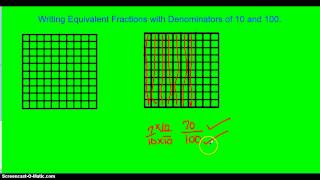By Randykidd

Using Equivalent Fractions to add two fractions with denominators of 10 and 100# Finding Equivalent Fractions using a Bar Model

##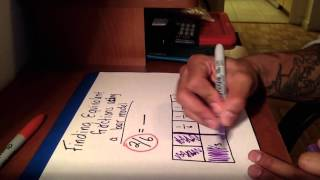By Math with Mr.OK

Explain equivalence of fractions in special cases, and compare fractions by reasoning about their size# Unit Fractions and Multiples of Fractions

##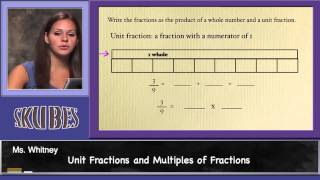By Skubes ed

Understand a multiple of a/b as a multiple of 1/b, and use this understanding to multiply a fraction by a whole number.# Plot Fractions on the Number Line

##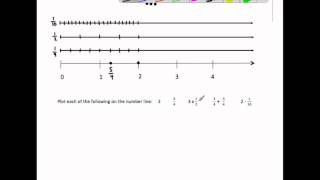By MathwithMrAlmeida

This video plots 6 fractions and operations with fractions on the number line. It ends by plotting all the same fractions on the number line and identify a pair of equivalent fractions and comparing each number's distance from zero.# Comparing fractions | Fractions | Pre-Algebra | Khan Academy

##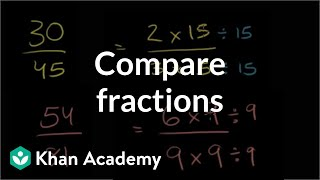By Khan Academy

Comparing Fractions# Comparing Fractions

##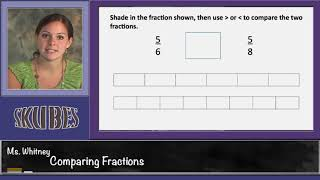By Skubes ed

Explain equivalence of fractions in special cases, and compare fractions by reasoning about their size.# Unit Fractions and Multiples of Fractions

##By Skubes ed

Understand a fraction a/b as a multiple of 1/b# Renaming Fractions and Mixed Numbers

##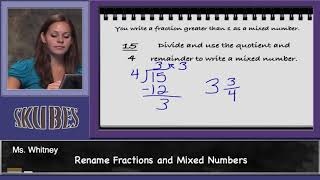By Skubes ed

Decompose a fraction into a sum of fractions with the same denominator in more than one way, recording each decomposition by an equation. Justify decompositions# Writing Fractions as Decimals

##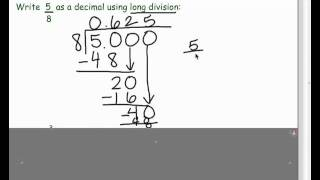By Mathademics

Learn how to write fractions as decimals.# Comparing fractions with different denominators

##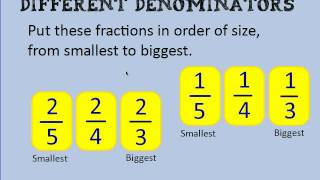By KSAMathematics

Comparing fractions with different denominators and different numerators# Comparing Fractions With Different Denominators

##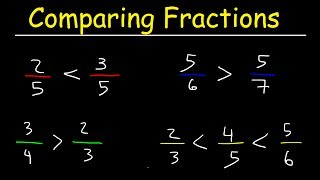By The Organic Chemistry Tutor

explains how to compare fractions with unlike / different denominators.